Feeds:
Posts

# Infinitary logic

Those unfamiliar with mathematical logic or the concept of ordinals are advised to consult those articles first.

An infinitary logic is a logic that allows infinitely long statements and/or infinitely long proofs. Some infinitary logics may have different properties from those of standard first-order logic. In particular, infinitary logics may fail to be compact or complete. Notions of compactness and completeness that are equivalent in finitary logic sometimes are not so in infinitary logic. So for infinitary logics the notions of strong compactness and strong completeness are defined. In this article we shall be concerned with Hilbert-type infinitary logics, as these have been extensively studied and constitute the most straightforward extensions of finitary logic. These are not, however, the only infinitary logics that have been formulated or studied.

Considering whether a certain infinitary logic named Ω-logic is complete promises to throw light on the continuum hypothesis.

##  A word on notation and the axiom of choice

As we are presenting a language with infinitely long formulae it is not possible to write expressions down as they should be written. To get around this problem we use a number of notational conveniences which strictly speaking are not part of the formal language we are defining. We useto indicate an expression that is infinitely long. Where it is not clear the length of the sequence is noted afterwards. Where this notation becomes ambiguous or confusing we use suffixes such asto indicate an infinite disjunction over a set of formulae of cardinality δ. The same notation may be applied to quantifiers for example. This is meant to represent an infinite sequence of quantifiers for each Vγ where γ < δ.

All usage of suffices andare not part of formal infinitary languages. We assume the axiom of choice (as is often done when discussing infinitary logic) as this is necessary to have sensible distributivity laws.

##  Definition of Hilbert-type infinitary logics

A first-order infinitary logic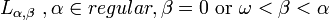has the same set of symbols as a finitary logic and may use all the rules for formation of formulae of a finitary logic together with some additional ones:

• If we have a set of variables V = {Vγ | γ < δ < β} and a formulae A0 thenandare formulae (In each case the sequence of quantifiers has length δ).
• If we have a set of formulae A = {Aγ | γ < δ < α} then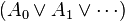andare formulae (In each case the sequence has length δ).

The concepts of bound variables apply in the same manner to infinite sentences. Note that the number of brackets in these formulae is always finite. Just as in finitary logic, a formula all of whose variables are bound is referred to as a sentence.

A theory T in infinitary logic Lα,β is a set of statements in the logic. A proof in infinitary logic from a theory T is a sequence of statements of length γ which obeys the following conditions: Each statement is either a logical axiom, an element of T, or is deduced from previous statements using a rule of inference. As before, all rules of inference in finitary logic can be used, together with an additional one:

• If we have a set of statements A = {Aγ | γ < δ < α} which have occurred previously in the proof then the statement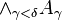can be inferred.

We give only those logical axiom schemata specific to infinitary logic. For each δ and γ such that 0 < α < δ we have the following logical axioms:

•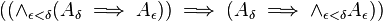• For each γ < δ we have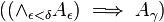• Chang’s distributivity laws (for each γ):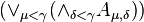whereand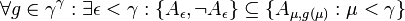• For γ < α we have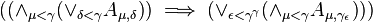where γε is a well ordering of γγ

The last two axiom schemata require the axiom of choice because certain sets must be well orderable. The last axiom schema is strictly speaking unnecessary as Chang’s distributivity laws imply it, however it is included as a natural way to allow natural weakenings to the logic.

##  Completeness, compactness and strong completeness

A theory is any set of statements. The truth of statements in models are defined by recursion and will agree with the definition for finitary logic where both are defined. Given a theory T a statement is said to be valid for the theory T if it is true in all models of T.

A logic Lα,β is complete if for every sentence S valid in every model there exists a proof of S. It is strongly complete if for any theory T for every sentence S valid in T there is a proof of S from T. An infinitary logic can be complete without being strongly complete.

A logic is compact if for every theory T of cardinality α if all subsets S of T have models then T has a model. A logic is strongly compact if for every theory T if all subsets S of T, where S has cardinality < α, have models then T has a model. If a logic is strongly compact, and complete, then it is strongly complete.

The cardinalis weakly compact if Lκ,κ is compact and κ is strongly compact if Lκ,κ is strongly compact.

##  Concepts expressible in infinitary logic

In the language of set theory the following statement expresses foundation:Unlike the axiom of foundation, this statement admits no non-standard interpretations. The concept of well foundedness can only be expressed in a logic which allows infinitely many quantifiers in an individual statement. As a consequence many theories, including Peano arithmetic, which cannot be properly axiomatised in finitary logic, can be in a suitable infinitary logic. Other examples include the theories of non-archimedean fields and torsion-free groups. These three theories can be defined without the use of infinite quantification; only infinite junctions are needed.

##  Complete infinitary logics

Two infinitary logics stand out in their completeness. These are Lω,ω and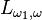. The former is standard finitary first-order logic and the latter is an infinitary logic that only allows statements of countable size.

Lω,ω is also strongly complete, compact and strongly compact.